# Pentaho/Contact Zone:MatchUp:Expression Elements

Overview
Tutorial
MatchUp Tabs
Matchcode
Field Mapping
Options
Source Pass-Through Columns
Lookup Pass-Through Columns
Output Filter
Expression Elements
Matchcodes
Result Codes

## Columns

The Columns section lists the columns available from the input.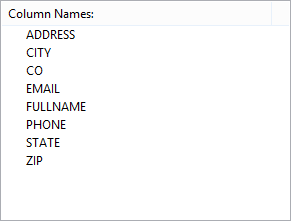## Variables

The Variables section lists the following system variables: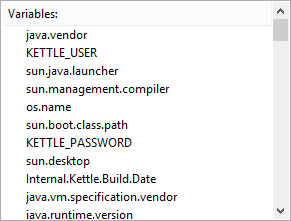Variable Name Data Type Description
ContainerStartTime DateTime The start time of the container.
CreationName String The name of the task.
LocaleID Int32 The locale that the task uses.
ParentContainerGUID String
CancelEvent Boolean Indicates whether the event handler stops running when an error, warning, or query cancellation occurs.
CreationDate DateTime The date that the package was created.
CreatorComputerName String The computer on which the package was created.
CreatorName String The name of the person who built the package.
ExecutionInstanceGUID String The unique identifier of the executing instance of a package.
FailedConfigurations String The names of package configurations that have failed.
InteractiveMode Boolean Indicates whether the package is run in interactive mode. If a package is running in the designer, this property is set to True. If a package is running using the DTExec command prompt utility, the property is set to False.
LastModifiedProductVersion
MachineName String The name of the computer on which the package is running.
OfflineMode Boolean Indicates whether the package is in offline mode. Offline mode does not acquire connections to data sources.
PackageID String The unique identifier of the package.
PackageName String The name of the package.
ProductVersion
StartTime DateTime The time that the package started to run.
UserName String The account of the user who started the package. The user name is qualified by the domain name.
VersionBuild Int32 The package version.
VersionGUID String The unique identifier of the version.
VersionMajor Int32 The major version of the package.
VersionMinor Int32 The minor version of the package.

## String Functions/Operators

The String Functions/Operators section lists the following functions/operators: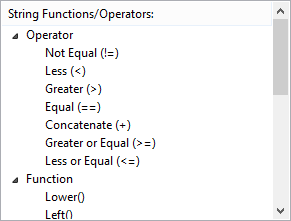### Operators

Operator Description
Concatenate (+) Concatenates two expressions.
Equal (==) Performs a comparison to determine if two expressions are equal.
Not Equal (!=) Performs a comparison to determine if two expressions are not equal.
Greater (>) Performs a comparison to determine if the first expression is greater than the second one.
Greater or Equal (>=) Performs a comparison to determine if the first expression is greater than or equal to the second one.
Less (<) Performs a comparison to determine if the first expression is less than the second one.
Less or Equal (<=) Performs a comparison to determine if the first expression is less than or equal to the second one.

### Functions

Function Description
CodePoint() Returns the Unicode code value of the leftmost character of a character expression.
FindString() Returns the one-based index of the specified occurrence of a character string within an expression.
Hex() Returns a string representing the hexadecimal value of an integer.
Len() Returns the number of characters in a character expression.
Left() Returns part of a character string starting at a specified number of characters from the left.
Lower() Returns a character expression after converting uppercase characters to lowercase characters.
LTrim() Returns a character expression after removing leading spaces.
PadLeft() Retruns a character expression after padding a specified string to the left of a specified character.
PadRight() Retruns a character expression after padding a specified string to the right of a specified character.
Replace() Returns a character expression after replacing a string within the expression with either a different string or an empty string.
Replicate() Returns a character expression, replicated a specified number of times.
Reverse() Returns a character expression in reverse order.
Right() Returns part of a character string starting at a specified number of characters from the right.
RTrim() Returns a character expression after removing trailing spaces.
SubString() Returns a part of a character expression.
Trim() Returns a character expression after removing leading and trailing spaces.
Upper() Returns a character expression after converting lowercase characters to uppercase characters.

## Numeric Functions/Operators

The Numeric Functions/Operators section lists the following functions/operators: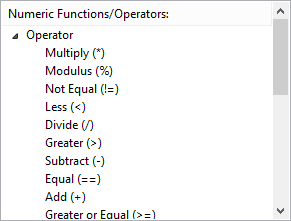### Operators

Operator Description
Subtract (-) Subtracts the second numeric expression from the first one.
Multiply (*) Multiplies two numeric expressions.
Divide (/) Divides the first numeric expression by the second one.
Modulus (%) Provides the integer remainder after dividing the first numeric expression by the second one.
Bitwise OR (|) Performs a bitwise OR operation of two integer values.
Bitwise AND (&) Performs a bitwise AND operation of two integer values.
Bitwise XOR (^) Performs a bitwise exclusive OR operation of two integer values.
Bitwise NOT (~) Performs a bitwise negation of an integer.
Equal (==) Performs a comparison to determine if two expressions are equal.
Not Equal (!=) Performs a comparison to determine if two expressions are not equal.
Greater (>) Performs a comparison to determine if the first expression is greater than the second one.
Greater or Equal (>=) Performs a comparison to determine if the first expression is greater than or equal to the second one.
Less (<) Performs a comparison to determine if the first expression is less than the second one.
Less or Equal (<=) Performs a comparison to determine if the first expression is less than or equal to the second one.

### Functions

Function Description
Abs() Returns the absolute, positive value of a numeric expression.
Exp() Returns the exponent to base e of the specified expression.
Ceiling() Returns the smallest integer that is greater than or equal to a numeric expression.
Floor() Returns the largest integer that is less than or equal to a numeric expression.
Ln() Returns the natural logarithm of a numeric expression.
Log() Returns the base-10 logarithm of a numeric expression.
Power() Returns the result of raising a numeric expression to a power.
Round() Returns a numeric expression that is rounded to the specified length or precision.
Sign() Returns the positive (+), negative (-), or zero (0) sign of a numeric expression.
Sqrt() Returns the square root of a numeric expression.
Square() Returns the square of a numeric expression.

## Boolean Functions/Operators

The Boolean Functions/Operators section lists the following functions/operators: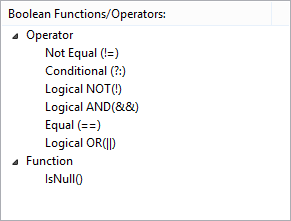### Operators

Operator Description
Logical OR (||) Performs a logical OR operation.
Logical AND (&&) Performs a logical AND operation.
Logical NOT (!) Negates a Boolean operand.
Equal (==) Performs a comparison to determine if two expressions are equal.
Not Equal (!=) Performs a comparison to determine if two expressions are not equal.
Conditional (?:) Returns one of two expressions based on the evaluation of a Boolean expression.

### Functions

Function Description
IsNull() Returns a Boolean result based on whether an expression is null.

## Date/Time Functions/Operators

The Date/Time Functions/Operators section lists the following functions/operators: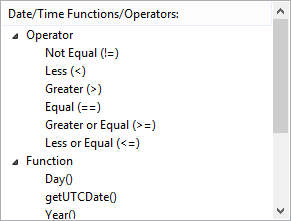### Operators

Operator Description
Equal (==) Performs a comparison to determine if two expressions are equal.
Not Equal (!=) Performs a comparison to determine if two expressions are not equal.
Greater (>) Performs a comparison to determine if the first expression is greater than the second one.
Greater or Equal (>=) Performs a comparison to determine if the first expression is greater than or equal to the second one.
Less (<) Performs a comparison to determine if the first expression is less than the second one.
Less or Equal (<=) Performs a comparison to determine if the first expression is less than or equal to the second one.

### Functions

Function Description
DateAdd() Returns a new DT_DBTIMESTAMP value by adding a date or time interval to a specified date.
DateDiff() Returns the number of date and time boundaries crossed between two specified dates.
DatePart() Returns an integer representing a datepart of a date.
Day() Returns an integer that represents the day of the specified date.
GetDate() Returns the current date of the system.
GetUTCDate() Returns the current date of the system in UTC time (Universal Time Coordinate or Greenwich Mean Time).
Month() Returns an integer that represents the month of the specified date.
Year() Returns an integer that represents the year of the specified date.

## Misc. Functions/Operators

The Misc. Functions/Operators section lists the following functions/operators: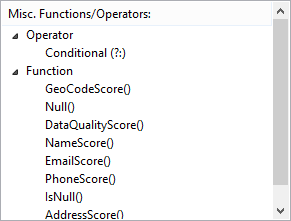### Operators

Operator Description
Conditional(?:) Returns one of two expressions based on the evaluation of a Boolean expression.

### Functions

Function Description
DataQualityScore() Returns a quality score for the results codes.
EmailScore() Returns a quality score for the email results codes.
GeoCodeScore() Returns a quality score for the geocode results codes.
IsNull() Returns a Boolean result based on whether an expression is null.
NameScore() Returns a quality score for the name results codes.
Null() Returns a null value of a requested data type.
PhoneScore() Returns a quality score for the phone results codes.

## DataType Casts

The DataType Casts section lists the following system variables: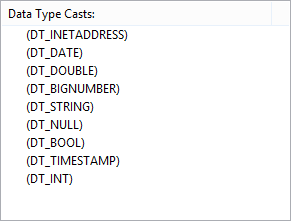Data Type Description
DT_STR A null-terminated ANSI/MBCS character string with a maximum length of 8000 characters. (If a column value contains additional null terminators, the string will be truncated at the occurrence of the first null.)
DT_WSTR A null-terminated Unicode character string with a maximum length of 4000 characters. (If a column value contains additional null terminators, the string will be truncated at the occurrence of the first null.)
DT_TEXT An ANSI/MBCS character string with a maximum length of 231-1 (2,147,483,647) characters.
DT_NTEXT A Unicode character string with a maximum length of 230 - 1 (1,073,741,823) characters.
DT_I1 A one-byte, signed integer.
DT_I2 A two-byte, signed integer.
DT_I4 A four-byte, signed integer.
DT_I8 An eight-byte, signed integer.
DT_UI1 A one-byte, unsigned integer.
DT_UI2 A two-byte, unsigned integer.
DT_UI4 A four-byte, unsigned integer.
DT_UI8 An eight-byte, unsigned integer.
DT_R4 A single-precision floating-point value.
DT_R8 A double-precision floating-point value.
DT_CY A currency value. This data type is an eight-byte signed integer with a scale of 4 and a maximum precision of 19 digits.
DT_DECIMAL An exact numeric value with a fixed precision and a fixed scale. This data type is a 12-byte unsigned integer with a separate sign, a scale of 0 to 28, and a maximum precision of 29.
DT_NUMERIC An exact numeric value with a fixed precision and scale. This data type is a 16-byte unsigned integer with a separate sign, a scale of 0 - 38, and a maximum precision of 38.
DT_BOOL A Boolean value.
DT_DATE A date structure that consists of year, month, day, hour, minute, seconds, and fractional seconds. The fractional seconds have a fixed scale of 7 digits.

The DT_DATE data type is implemented using an 8-byte floating-point number. Days are represented by whole number increments, starting with 30 December 1899, and midnight as time zero. Hour values are expressed as the absolute value of the fractional part of the number. However, a floating point value cannot represent all real values; therefore, there are limits on the range of dates that can be presented in DT_DATE. On the other hand, DT_DBTIMESTAMP is represented by a structure that internally has individual fields for year, month, day, hours, minutes, seconds, and milliseconds. This data type has larger limits on ranges of the dates it can present.

DT_DBDATE A date structure that consists of year, month, and day.
DT_DBTIME A time structure that consists of hour, minute, and second.
DT_DBTIME2 A time structure that consists of hour, minute, second, and fractional seconds. The fractional seconds have a maximum scale of 7 digits.
DT_DBTIMESTAMP A timestamp structure that consists of year, month, day, hour, minute, second, and fractional seconds. The fractional seconds have a fixed scale of 3 digits.
DT_DBTIMESTAMP2 A timestamp structure that consists of year, month, day, hour, minute, second, and fractional seconds. The fractional seconds have a maximum scale of 7 digits.
DT_DBTIMESTAMPOFFSET A timestamp structure that consists of year, month, day, hour, minute, second, and fractional seconds. The fractional seconds have a maximum scale of 7 digits.

Unlike the DT_DBTIMESTAMP and DT_DBTIMESTAMP2 data types, the DT_DBTIMESTAMPOFFSET data type has a time zone offset. This offset specifies the number of hours and minutes that the time is offset from the Coordinated Universal Time (UTC). The time zone offset is used by the system to obtain the local time. The time zone offset must include a sign, plus or minus, to indicate whether the offset is added or subtracted from the UTC. The valid number of hours offset is between -14 and +14. The sign for the minute offset depends on the sign for the hour offset: If the sign of the hour offset is negative, the minute offset must be negative or zero. If the sign for the hour offset is positive, the minute offset must be positive or zero. If the sign for the hour offset is zero, the minute offset can be any value from negative 0.59 to positive 0.59.

DT_FILETIME A 64-bit value that represents the number of 100-nanosecond intervals since January 1, 1601. The fractional seconds have a maximum scale of 3 digits.